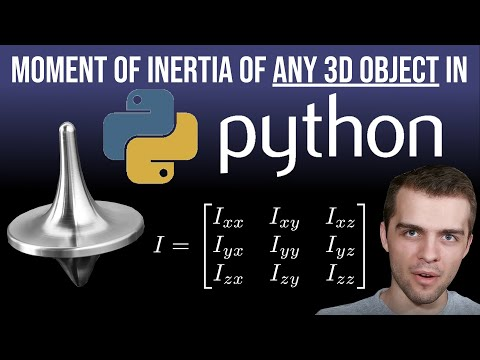# Moment of Inertia For ANY 3D Object In PythonIn this video I find the moment of inertia for 3D objects in two different ways. In the first technique, I define a 3D object mathematically and evaluate integrals to get the moment of inertia tensor. In the second technique, I load the coordinates of a general 3D object and evaluate the tensor that way.

In this video I find the moment of inertia for 3D objects in two different ways. In the first technique, I define a 3D object mathematically and evaluate integrals to get the moment of inertia tensor. In the second technique, I load the coordinates of a general 3D object and evaluate the tensor that way.

0:00 Introduction
2:05 Define 3D Object Mathematically